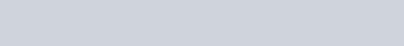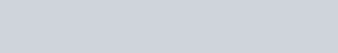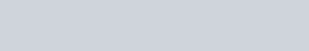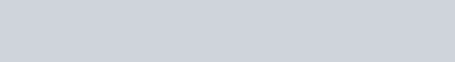Application of Le Chatelier's Principle In Chemical Reactions - GulpMatrix

# Application of Le Chatelier’s Principle In Chemical ReactionsIn explaining the significance of the Le chatelier’s principle, we shall first have a brief look at what a reversible reaction is. Firstly, every reversible reaction reaches its own specific equilibrium under a given set of conditions. This equilibrium state is dependent on

• Temperature of the reacting system;
• Pressure of the reacting system (for gases);
• Concentration of the reacting system.

A change in any one of these factors will upset the balance of the system and result in a shift in the position of the equilibrium. These factors and their effects on chemical systems in equilibrium were studied by Le Chatelier (1850 – 1936) who formulated the Le Chatelier’s Principle.

Le Chatelier’s Principle states that if an external constraint such as a change in temperature, pressure or concentration, is imposed on a chemical system in equilibrium, the equilibrium will shift so as to annul or neutralize the constraint.

The Le Chatelier’s principle is of great importance in chemical industry because it can help to

• Define the optimum conditions for the chemical processes employed in industry;
• Reduce undesirable reversibility;
• Predict the effect of an altered factor on the equilibrium position of an untried reaction.

Effect Of A Change In Temperature

In the following thermochemical equations,

A + B          C                 ΔH = +ve

X + Y         Z                 ΔH = -ve

• If ΔH is positive, the forward reaction is endothermic, and the backward reaction is exothermic;
• If ΔH is negative, the forward reaction is exothermic, and the backward reaction is endothermic.

If a chemical system is in equilibrium and the temperature is lowered, then according to Le Chatelier’s Principle, the equilibrium position will shift so as to annul the cooling effect by releasing more heat. Conversely, if the temperature is raised, then the equilibrium position will shift so as to annul the heating effect by absorbing more heat. In the following reversible reaction, the forward reaction is endothermic.

N2(g) + O2(g)        ΔH = -395.7KJ mol-1

• Increasing the temperature of the system will shift the equilibrium position to the right, favouring the forwards reaction, i.e., product formation. Hence, the value of the equilibrium constant K also increases. So, for a given reversible reaction, a higher value of K would mean a greater yield of product(s).
• Lowering the temperature of the system will shift the equilibrium position to the left, favouring the backward reaction, i.e., reactant formation. This results in a lowering of K So, for a given reversible reaction a lower value of K would mean a greater yield of reactant(s).

In the following reaction, the forward reaction is exothermic

2SO2(g) + O2(g)   =       2SO3(g)       ΔH = -395.7KJ mol-1

In this case, an increase in temperature will cause the equilibrium position to shift to the left, i.e., it favours reactant formation. Conversely, a decrease in temperature will cause the equilibrium position to shift to the right, i.e., it favours product formation.

Generally, according to Le Chatelier’s Principle, the forward reaction in an exothermic system is favoured by lowering the temperature, while the forward reaction of an endothermic system is favoured by raising the temperature.

We can illustrate the effect of temperature changes on equilibrium position with the nitrogen(IV) oxide (N2O4) nitrogen(IV) oxide (NO2) chemical system.

Dinitrogen(IV) oxide molecules are pale yellow, whereas nitrogen(IV) oxide molecules are reddish-brown in colour. At low temperatures, dinitrogen(IV) oxide predominates while at higher temperatures, it dissociates to give the monomer. NO2. If a sealed glass vessel containing an equilibrium mixture of the two is warmed or cooled, the colour changes give a visual demonstration of the effect of temperature on the equilibrium position of the reaction.Effect Of A Change In Pressure

For a change in pressure to affect a chemical system in equilibrium,

• One of the reactants or products in the reversible reaction must be gaseous;
• The total number of moles of gaseous molecules on the left side of the equation must be different from the total number of moles of gaseous molecules on the right side.

An example of such a reaction is the formation of ammonia from nitrogen and hydrogen. Here, 4 moles (or volumes) of reactants give only 2 moles (or volumes) of products. The forward reaction results in a decrease in the pressure of the system. The backward reaction results in an increase in the number of moles and hence an increase in the pressure of the system.According to Le Chatelier’s Principle, if a high pressure is applied to an equilibrium system, the reaction which involves a reduction in pressure will be favored. Conversely, if low pressure is imposed on an equilibrium system, then the reaction which results in an increase in pressure will be favoured.

In the formation of ammonia, a high pressure will favour the forward reaction where a reduction in volume occurs, causing the equilibrium position to shift to the right. This is because the forward reaction will counteract the high pressure imposed on the system by lowering the pressure, thus keeping the equilibrium constant, K, unchanged. In the process, a high percentage yield of the product is obtained

On the other hand, a low pressure will cause the equilibrium position of this system to shift to the left, favouring the backward reaction. This will cause an increase in the pressure of the system and, thus, keep the equilibrium constant unchanged. It will also result in high yields of the reactants.

In the following endothermic reaction, the forward reaction results in an increase in volume.

N2O4(g)   =    2NO(g)

In this case, a low pressure will favor the forward reaction and product formation, while a high pressure will favor the backward reaction.

In reversible reactions involving the same number of moles of the equation as shown in the reaction below, a change in pressure has no effect on the position of equilibrium. However, the rate at which equilibrium is attained is faster at higher pressures.Effect Of A Change In Concentration

In an equilibrium mixture, there is a balance between the concentrations of the reactants and the products, i.e., these concentrations are in a definite ratio which depends on the conditions of the reaction. If more reactants are introduced into the equilibrium system, the balance will be upset. In order to relieve this constraint (i.e., the increase in the concentration of reactants), the equilibrium position will shift to the right, favouring the forward reaction. This results in a proportional increase in the concentration of the products, and so the equilibrium constant remains unchanged. Another way of increasing the amount of products formed is by continually removing the products from the system. Again, in order to relieve the constraint, the equilibrium will shift to the right, favouring the forward reaction.

In the reversible reaction of iron with steam,The hydrogen is constantly being removed by a current of steam that passes over the heated iron, until all the iron is converted to iron (II) diiron (III) oxide. The reverse process occurs if water vapor is removed.

The preparation of many important chemicals from reversible systems depends upon the principle of removing one of the products from the reaction vessel. In the manufacture of calcium oxide by the heating of limestone in a limekiln, the carbon (IV) oxide produced is removed by an air current.We can illustrate the effect of concentration on the position of equilibrium by using the following chemical systems.

Iron (III) tricyanate system: When we add some iron (III) trioxonitrate (V) solution to an equal volume of potassium thiocyanate solution, a blood-red colouration of a certain intensity results when equilibrium is reached. The reaction is represented as follows:The intensity of the red colour in the reaction mixture remains the same as long as the equilibrium is not disturbed. If the concentration of a reactant or product is changed, the equilibrium position shifts to annul the change. This is seen by a change in the colour intensity of the reaction mixture.

Potassium heptaoxochromate (VI) system: Potassium heptaoxochromate (VI) is an orange colored solid which when dissolved in water, dissociates into hydrogen ions and yellow tetraoxochromate(VI) ions as shown.At neutral pH, there are more teraoxochromate (VI) ions than heptaoxochromate(VI) ions. The yellow color of the tetraoxochromate (VI) ions masks the orange color of the heptaoxochromate(VI) ions. Thus, a neutral solution of potassium heptaoxochromate(VI) looks yellow.

When the hydrogen ion concentration is increased (by adding an acid), the equilibrium position shifts to the left. This is reflected by the colour of the solution changing from yellow to orange. When the hydrogen ion concentration is decreased (by adding an alkali), the equilibrium position shifts to the right as indicated by an increase in the intensity of the yellow colour.

Effect Of Catalyst

We have learnt that a catalyst does not change the position of equilibrium. Thus, it cannot be used to increase product formation. However, catalysts are extremely important in chemical industry because they affect the time taken for a reaction to reach equilibrium. A positive catalyst increases the rates of both the forward and backward reactions to reach equilibrium. A positive catalyst increases the rates of both the forward and backward reactions to the same extent by lowering the activation energy of the reaction. As a result, equilibrium is reached in a shorter time than in an uncatalyzed reaction.

A negative catalyst slows down the rate of reaction so that a longer time is needed to reach equilibrium.